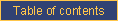homefeaturesexamplesDOCUMENTATIONblogpricingcontact ustechnical documentationCATI documentationcookbookrobotDebugging a complex calculation

Problem

You need to determine why a calculation does not work.

Solution

Use "print" commands in an anonymous subroutine structure.

Discussion

We realize this discussion is technical; so are complex calculations.

Most of the problems with complex calculations have to do with the absence of the dollar sign as prefix to variable names (required in the Perl context), with variables not being properly initialized and with improper mathematical syntax. Therefore, use the following debugging guidelines.

• make sure that each variable (or question) name is prefixed with a dollar sign. The following DOES NOT WORK: Q2 = Q1+2 but this does work: Q2 = \$Q1+2
• "print" the value of key variables used using the following syntax (put the entire calculation within braces and end each statement with a semi-colon):
Q2 =
{
print "\\$Q1 = \$Q1";
return \$Q1+2
}
• in the case of a complex expression, break it up and print intermediate results, such as:
Q5 =
{
print "\\$Q1 = \$Q1<BR>\\$Q2 = \$Q2<BR>\\$Q3 = \$Q3<BR>";
print "Q1+Q2 = ".(\$Q1 + \$Q2)."<BR>";
return \$Q1+\$Q2+\$Q3
}e-mail technical support!